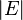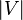# Graph (mathematics) facts for kids

Kids Encyclopedia Facts

In mathematics, a graph is used to show how things are connected. The things being connected are called vertices, and the connections among them are called edges. If vertices are connected by an edge, they are called adjacent. The degree of a vertex is the number of edges that connect to it. A graph's size$|E|$is the number of edges in total. The number of vertices, written as$|V|$, is called the order of a graph.

Edges may have weights, which show the costs associated with using each edge. In a graph of cities on a map, the cost may be the distance between two cities, or the amount of time it takes to travel between the two.

Directed graphs go in one direction, like water flowing through a bunch of pipes. Undirected graphs don't have a direction, like a mutual friendship. A graph where there is more than one edge between two vertices is called multigraph. One where there is at most one edge is called a simple graph. A simple graph, where every vertex is directly connected to every other is called complete graph. A loop is an edge that connects to its own vertex. Loops are only allowed in multigraphs.

A sequence of edges is called a path. A path which starts and ends at the same edge is called a cycle.Graph (mathematics) Facts for Kids. Kiddle Encyclopedia.